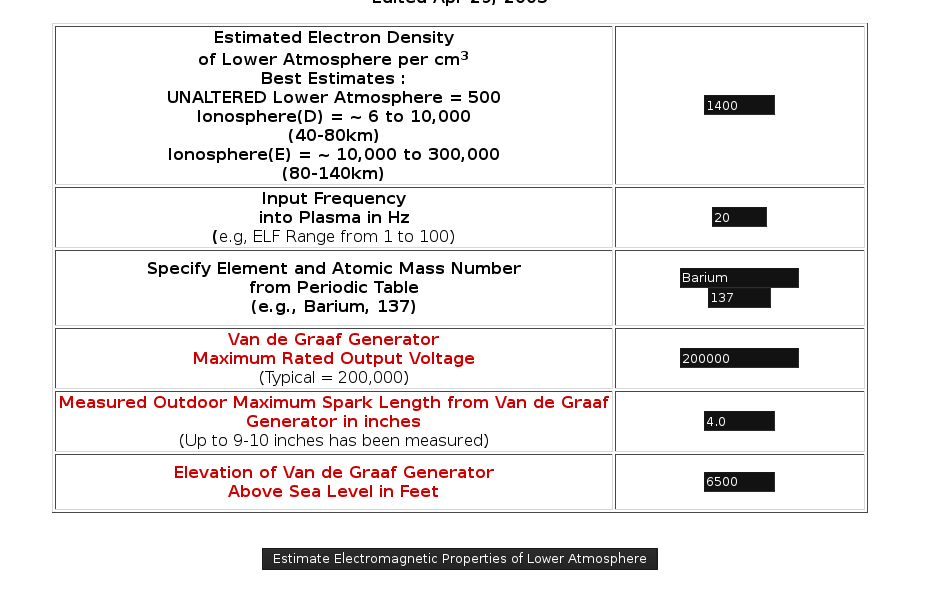ESTIMATED LOWER ATMOSPHERIC
ELECTROMAGNETIC PROPERTIES
Clifford E Carnicom
Santa Fe, NM
May 11 2003
Edited Apr 29, 2005

Expected conductivity of lower atmosphere
is now increased by an estimated factor of 3 to 20,
depending upon atmospheric and aerosol conditions.

Note resonant ELF cyclotron frequency of barium
as well as most physiologically important ions (e.g, Mg, Ca, K etc.).

Assumed Values or Constants for Computations1:

Speed of Light (c) : 3E8 m / sec
Mass of an electron (m) : 9.11E-31kg
Magnetic field strength of earth (B) : 5E-5 Tesla
Permeability of free space (uo) : 4 * pi * 1E-7
Electron charge (e) : -1.6E-19 coulombs
Permittivity of free space (eo) : 8.85E-12 C2 / N * m2
Boltzmann’s constant (kb) : 1.38E-23 J / K
Temperature of lower atmosphere (t) : 22 deg C.
Breakdown Voltage (Ebmax) (Dielectric Strength) of Normal Air = 3E6 Volts/meter
Work of Ionization of Atmosphere (Wion) = 5E-18 J (~30eV)
T = temperature of lower atmosphere in Kelvin
sigmanorm = conductivity of normal lower atmosphere : 2E-14 ohm-1

1. Predicted Whistler Frequency in Hertz (Hz)(Right Circular Polarization) Dispersion Relationship:2,3:

k = ( w / c) * ( 1 + ( wpe2 / ( w * (wce – w) ) ) ) 1/2

and

fhz = (k * c) / (2 * pi)

where

w = plasma input frequency in radians

c = speed of light

wpe = plasma frequency in radians

wce = cyclotron frequency of an electron in earth’s magnetic field

k = wave number

fhz = frequency in hertz.

2.
Predicted Afven Frequency in Hertz (Hz) Dispersion Relationship:4:

k = ( w / c) * ( 1 + ( ( ni * m * c2 ) / ( B2 / uo ) ) ) 1/2

ni = electron density per m3

m = mass of electron

B = strength of Earth’s magnetic field

uo = permeability of free space

3. Plasma Frequency in Radians5 :

wpe = ( ni * e2 ) / ( m * eo ) 1/2

eo = permittivity of free space

4. Debye Length6 :

rD = ( (kb * T) / m ) 1/2 * ( 1 / wpe)

T = temperature in degrees Kelvin

kb = Boltzmann’s constant

5. Plasma Parameter7 :

ND = ( (4 * pi ) / 3) * rD3 * ni

6. Cyclotron Frequency in hertz8 :

fg = 1.54E3 * (Bgauss / A )

where A = the mass number of the ion.

Bgauss = earth magnetic field strength in gauss.

7.
Estimated Lower Atmospheric Conductivity Ratio Estimate (based upon linear relationship of conductivity with n). (METHOD 1):

sigma_ratioest = niest / ninormal

where ninormal is the normal expected electron density per cm3 of the lower atmosphere (~500) and niest is the estimated electron density of the lower atmosphere. This method requires a knowledge of electron density, and is therefore difficult to achieve.

8.
Estimated Lower Atmospheric Conductivity Ratio Estimate – based upon linear relationship of conductivity to the spark length and the exponential relationship of electron generation under breakdown conditions. (METHOD 2):

sigma_ratioest =(( ealpha * dmeas) – 1 ) / (( ealpha * dcalc) – 1 ) * ( dmeas / dcalc )

where alpha is the first Townsend coefficient in units of 1 / (m * torr ) as is modeled by the following developed equation:

alpha = 4.68E-8 * ( Ebmax / ( 762 * e-.00004h) )2.21

where h is the elevation of the Van de Graaf generator above sea level in feet. This equation is developed from a least squares analysis in conjunction with the listed references15,16,18.

In addition, dmeas is the measured spark length of the Van de Graaf generator in meters within the modified atmosphere and dcalc is the theoretical spark length of the Van de Graaf generator of the normal atmosphere.

This work has been developed using a series of references15, 16, 17, and will be explained in more detail on a separate page related to conductivity investigations and analysis. This method requires only measurements that are available with the use of the Van de Graaf generator.

9. Cyclotron resonant frequency of an electron9 :

wce = ( ( e * B ) / m )

10. Atmospheric Conductivity ( Method 1):

sigma = (niest / ninormal) * sigmanorm

11. Atmospheric Conductivity ( Method 2):

Refer to page entitled Atmospheric Conductivity II.

References:

1. Gordon J. Coleman, The Addison-Wesley Science Handbook (Addison-Wesley Publishers Limited, 1997)
2. R.O. Dendy, Plasma Dynamics (Oxford University Press, 2000), 41.
3. William C. Elmore, Physics of Waves (Dover, 1985), 10.
4. Dendy, 51.
5. Dendy, 7.
6. Dendy, 10.
7. Dendy, 11.
8. United States Patent # 4686605, Bernard Eastlund, Method and Apparatus for Altering a Region in the Earth’s Atmosphere, Ionosphere, and/or Magnetosphere, 1987, 6.
9. Alan C. Tribble, Princeton Guide to Advanced Physics (Princeton University Press, 1996), 147.
10. Charles Papas, Theory of Electromagnetic Wave Propagation, (Dover, 1988), 177.
11. Niels Jonassen, Breakdown, Compliance Engineering, www.ce-mag.com/archive/01/01/MrStatic.html, (eq. 4).
12. Jonassen, ( eq. 10).
13. Michael Mansfield, Understanding Physics, (Wiley and Sons, 1998), 288.
14. Niels Jonassen, Environmental ESD, Part 1 : The Atmospheric Electric Circuit, Compliance Engineering, www.ce-mag.com/archive/02/07/mrstatic.html, (eq. 4).
15. Martin A. Ulman, Lightning, (Dover, 1984), 204-206.
16. Dwight E. Gray, Ph. D., American Institute of Physics Handbook, (McGraw-Hill Book Company, Inc., 1963), 5-138 to 5-140.
17. David R. Lide, CRC Handbook of Chemistry and Physics, 82ed, (CRC Press, 2001), 14-19 to 14-20.
18. Claude Irwin Palmer, Practical Calculus for Home Study, (McGraw-Hill Book Company, 1924), 151.